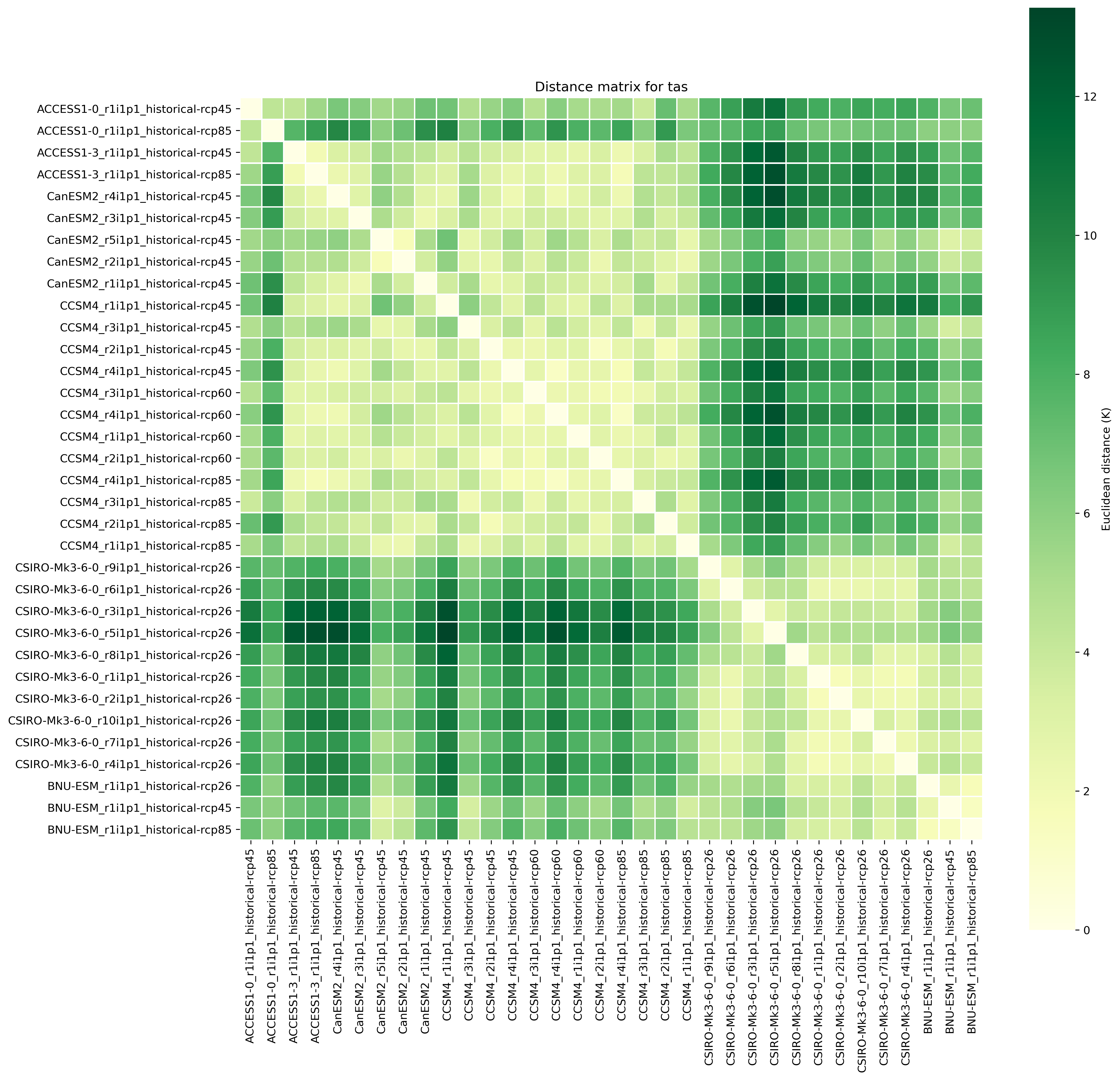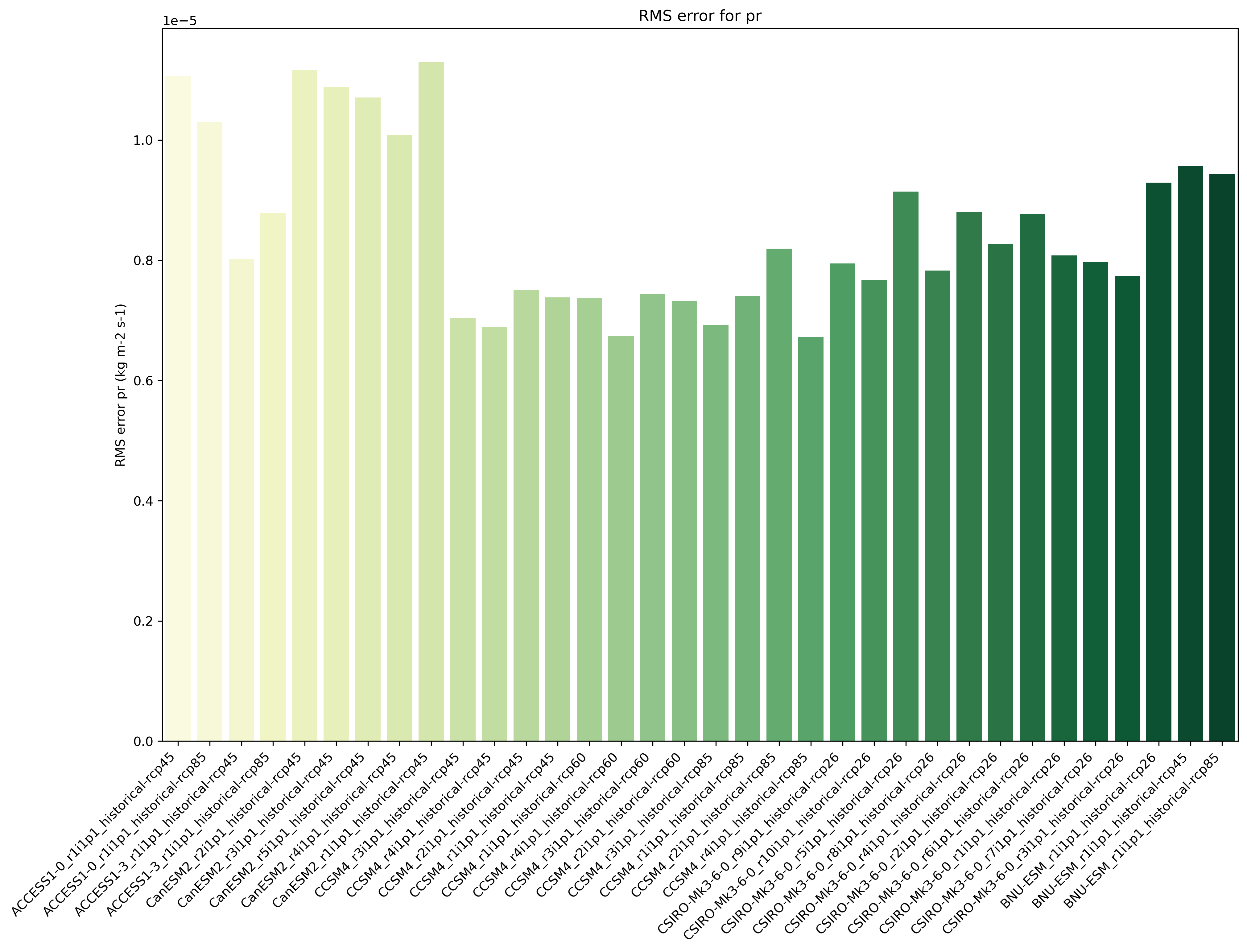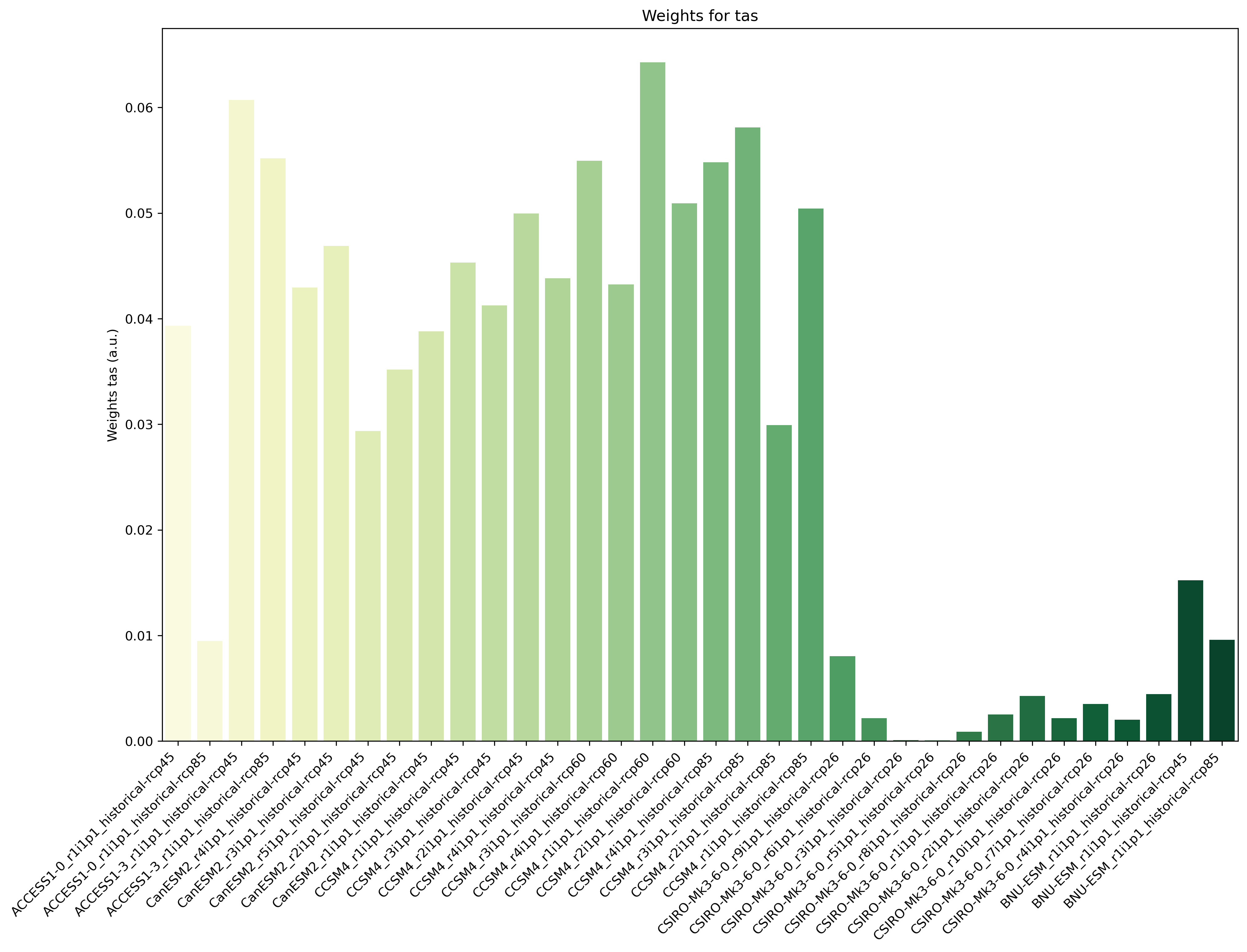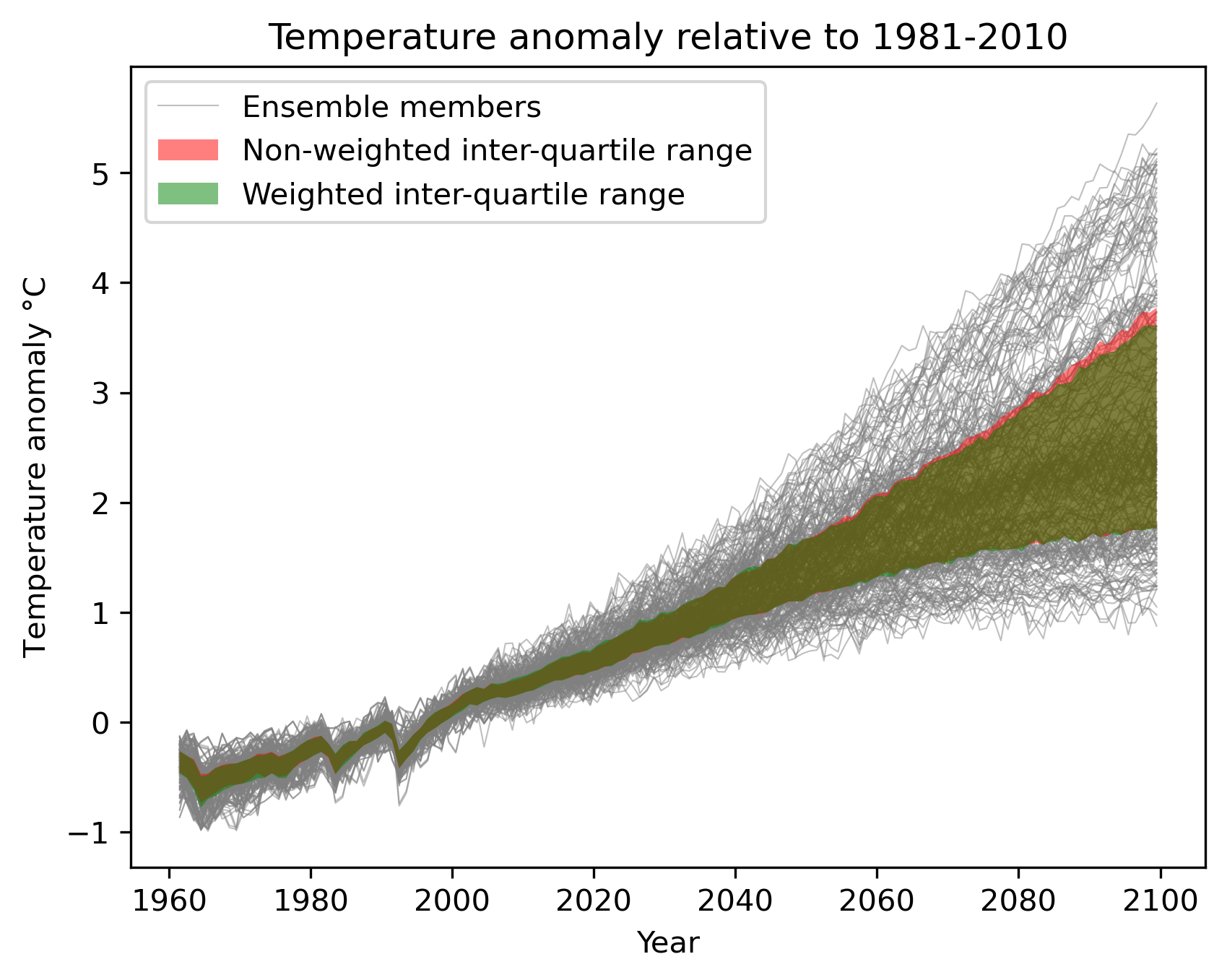# ClimWIP: independence & performance weighting¶

## Overview¶

This recipe calculates weights based on combined performance and independence metrics. These weights can be used in subsequent diagnostics. Reference implementation based on https://github.com/lukasbrunner/ClimWIP

## Available recipes and diagnostics¶

Recipes are stored in esmvaltool/recipes/

• recipe_climwip.yml

Diagnostics are stored in esmvaltool/diag_scripts/weighting/

• climwip.py: Compute weights for each input dataset

• weighted_temperature_graph.py: Show the difference between weighted and non-weighted temperature anomalies.

## User settings in recipe¶

1. Script climwip.py

Required settings for script
• sigma_performance: shape parameter weights calculation (determined offline)

• sigma_independence: shape parameter for weights calculation (determined offline)

• obs_data: list of project names to specify which are the the observational data. The rest is assumed to be model data.

Required settings for variables
• This script takes multiple variables as input as long as they’re available for all models

• start_year: provide the period for which to compute performance and independence.

• end_year: provide the period for which to compute performance and independence.

• mip: typically Amon

• preprocessor: e.g. climwip_summer_mean

• additional_datasets: provide a list of model data for performance calculation.

Optional settings for variables
• performance: set to false to not calculate performance for this variable group

• independence: set to false to not calculate independence for this variable group

• By default, both performance and independence are calculate for each variable group.

Required settings for preprocessor
• Different combinations of preprocessor functions can be used, but the end result should always be aggregated over the time dimension, i.e. the input for the diagnostic script should be 2d (lat/lon).

Optional settings for preprocessor
• extract_region or extract_shape can be used to crop the input data.

• extract_season can be used to focus on a single season.

• different climate statistics can be used to calculate mean or (detrended) std_dev.

1. Script weighted_temperature_graph.py

Required settings for script
• ancestors: must include weights from previous diagnostic

• weights: the filename of the weights: ‘weights_combined.nc’

Required settings for variables
• This script only takes temperature (tas) as input

• start_year: provide the period for which to plot a temperature change graph.

• end_year: provide the period for which to plot a temperature change graph.

• mip: typically Amon

• preprocessor: temperature_anomalies

Required settings for preprocessor
• Different combinations of preprocessor functions can be used, but the end result should always be aggregated over the latitude and longitude dimensions, i.e. the input for the diagnostic script should be 1d (time).

Optional settings for preprocessor
• Can be a global mean or focus on a point, region or shape

• Anomalies can be calculated with respect to a custom reference period

• Monthly, annual or seasonal average/extraction can be used

## Variables¶

• pr (atmos, monthly mean, longitude latitude time)

• tas (atmos, monthly mean, longitude latitude time)

• more variables can be added if available for all datasets.

## Observations and reformat scripts¶

Observation data is defined in a separate section in the recipe and may include multiple datasets.

## Example plots¶Fig. 4 Distance matrix for temperature, providing the independence metric.Fig. 5 Distance of preciptation relative to observations, providing the performance metric.Fig. 6 Weights determined by combining independence and performance metrics for tas.Fig. 7 Interquartile range of temperature anomalies relative to 1981-2010, weighted versus non-weighted.